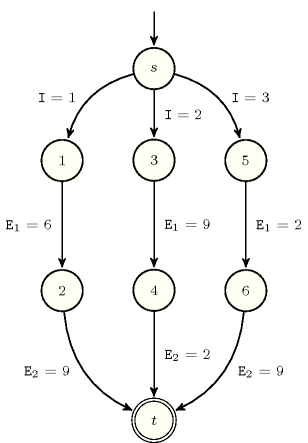## 5.145. elementn

Origin

P. Flener

Constraint

$\mathrm{𝚎𝚕𝚎𝚖𝚎𝚗𝚝𝚗}\left(\mathrm{𝙸𝙽𝙳𝙴𝚇},\mathrm{𝚃𝙰𝙱𝙻𝙴},\mathrm{𝙴𝙽𝚃𝚁𝙸𝙴𝚂}\right)$

Arguments
 $\mathrm{𝙸𝙽𝙳𝙴𝚇}$ $\mathrm{𝚍𝚟𝚊𝚛}$ $\mathrm{𝚃𝙰𝙱𝙻𝙴}$ $\mathrm{𝚌𝚘𝚕𝚕𝚎𝚌𝚝𝚒𝚘𝚗}\left(\mathrm{𝚟𝚊𝚕𝚞𝚎}-\mathrm{𝚒𝚗𝚝}\right)$ $\mathrm{𝙴𝙽𝚃𝚁𝙸𝙴𝚂}$ $\mathrm{𝚌𝚘𝚕𝚕𝚎𝚌𝚝𝚒𝚘𝚗}\left(\mathrm{𝚎𝚗𝚝𝚛𝚢}-\mathrm{𝚍𝚟𝚊𝚛}\right)$
Restrictions
 $\mathrm{𝙸𝙽𝙳𝙴𝚇}\ge 1$ $\mathrm{𝙸𝙽𝙳𝙴𝚇}\le |\mathrm{𝚃𝙰𝙱𝙻𝙴}|-|\mathrm{𝙴𝙽𝚃𝚁𝙸𝙴𝚂}|+1$ $|\mathrm{𝚃𝙰𝙱𝙻𝙴}|>0$ $|\mathrm{𝙴𝙽𝚃𝚁𝙸𝙴𝚂}|>0$ $|\mathrm{𝚃𝙰𝙱𝙻𝙴}|\ge |\mathrm{𝙴𝙽𝚃𝚁𝙸𝙴𝚂}|$ $\mathrm{𝚛𝚎𝚚𝚞𝚒𝚛𝚎𝚍}$$\left(\mathrm{𝚃𝙰𝙱𝙻𝙴},\mathrm{𝚟𝚊𝚕𝚞𝚎}\right)$ $\mathrm{𝚛𝚎𝚚𝚞𝚒𝚛𝚎𝚍}$$\left(\mathrm{𝙴𝙽𝚃𝚁𝙸𝙴𝚂},\mathrm{𝚎𝚗𝚝𝚛𝚢}\right)$
Purpose

$\forall i\in \left[1,|\mathrm{𝙴𝙽𝚃𝚁𝙸𝙴𝚂}|\right]:\mathrm{𝙴𝙽𝚃𝚁𝙸𝙴𝚂}\left[i\right].\mathrm{𝚎𝚗𝚝𝚛𝚢}=\mathrm{𝚃𝙰𝙱𝙻𝙴}\left[\mathrm{𝙸𝙽𝙳𝙴𝚇}+i-1\right].\mathrm{𝚟𝚊𝚕𝚞𝚎}$

Example
$\left(3,〈6,9,2,9〉,〈2,9〉\right)$

The $\mathrm{𝚎𝚕𝚎𝚖𝚎𝚗𝚝𝚗}$ constraint holds since its third argument $\mathrm{𝙴𝙽𝚃𝚁𝙸𝙴𝚂}=〈2,9〉$ is set to the subsequence starting at the third (i.e., $\mathrm{𝙸𝙽𝙳𝙴𝚇}=3$) item of the table $\mathrm{𝚃𝙰𝙱𝙻𝙴}=〈6,9,2,9〉$.

Typical
 $|\mathrm{𝚃𝙰𝙱𝙻𝙴}|>1$ $\mathrm{𝚛𝚊𝚗𝚐𝚎}$$\left(\mathrm{𝚃𝙰𝙱𝙻𝙴}.\mathrm{𝚟𝚊𝚕𝚞𝚎}\right)>1$ $|\mathrm{𝙴𝙽𝚃𝚁𝙸𝙴𝚂}|>1$
Symmetry

All occurrences of two distinct values in $\mathrm{𝚃𝙰𝙱𝙻𝙴}.\mathrm{𝚟𝚊𝚕𝚞𝚎}$ or $\mathrm{𝙴𝙽𝚃𝚁𝙸𝙴𝚂}.\mathrm{𝚎𝚗𝚝𝚛𝚢}$ can be swapped; all occurrences of a value in $\mathrm{𝚃𝙰𝙱𝙻𝙴}.\mathrm{𝚟𝚊𝚕𝚞𝚎}$ or $\mathrm{𝙴𝙽𝚃𝚁𝙸𝙴𝚂}.\mathrm{𝚎𝚗𝚝𝚛𝚢}$ can be renamed to any unused value.

Arg. properties

Suffix-extensible wrt. $\mathrm{𝚃𝙰𝙱𝙻𝙴}$.

Usage

The $\mathrm{𝚎𝚕𝚎𝚖𝚎𝚗𝚝𝚗}$ constraint is useful for extracting of subsequence of fixed length from a given sequence.

Reformulation

Let ${I}_{1}=\mathrm{𝙸𝙽𝙳𝙴𝚇},{I}_{2}=\mathrm{𝙸𝙽𝙳𝙴𝚇}+1,\cdots ,{I}_{|\mathrm{𝙴𝙽𝚃𝚁𝙸𝙴𝚂}|}=\mathrm{𝙸𝙽𝙳𝙴𝚇}+|\mathrm{𝙴𝙽𝚃𝚁𝙸𝙴𝚂}|-1$. The $\mathrm{𝚎𝚕𝚎𝚖𝚎𝚗𝚝𝚗}$$\left(\mathrm{𝙸𝙽𝙳𝙴𝚇},\mathrm{𝚃𝙰𝙱𝙻𝙴},〈\mathrm{𝚎𝚗𝚝𝚛𝚢}-{E}_{1},\mathrm{𝚎𝚗𝚝𝚛𝚢}-{E}_{2},\cdots ,\mathrm{𝚎𝚗𝚝𝚛𝚢}-{E}_{|\mathrm{𝙴𝙽𝚃𝚁𝙸𝙴𝚂}|}〉\right)$ constraint can be expressed in term of a conjunction of $|\mathrm{𝙴𝙽𝚃𝚁𝙸𝙴𝚂}|$ $\mathrm{𝚎𝚕𝚎𝚖𝚎𝚗𝚝}$ constraints of the form:

$\mathrm{𝚎𝚕𝚎𝚖𝚎𝚗𝚝}$$\left({I}_{1},\mathrm{𝚃𝙰𝙱𝙻𝙴},{E}_{1}\right)$,

$\mathrm{𝚎𝚕𝚎𝚖𝚎𝚗𝚝}$$\left({I}_{2},\mathrm{𝚃𝙰𝙱𝙻𝙴},{E}_{2}\right)$,

$\cdots$

$\mathrm{𝚎𝚕𝚎𝚖𝚎𝚗𝚝}$$\left(\mathrm{𝙸𝙽𝙳𝙴𝚇}+|\mathrm{𝙴𝙽𝚃𝚁𝙸𝙴𝚂}|-1,\mathrm{𝚃𝙰𝙱𝙻𝙴},{E}_{|\mathrm{𝙴𝙽𝚃𝚁𝙸𝙴𝚂}|}\right)$.

Figure 5.145.1 depicts the automaton associated with the $\mathrm{𝚎𝚕𝚎𝚖𝚎𝚗𝚝𝚗}$ constraint of the Example slot. Let $𝙸$ and ${𝙴}_{k}$ respectively denote the $\mathrm{𝙸𝙽𝙳𝙴𝚇}$ argument and the $\mathrm{𝚎𝚗𝚝𝚛𝚢}$ attribute of the ${k}^{th}$ item of the $\mathrm{𝙴𝙽𝚃𝚁𝙸𝙴𝚂}$ collection. Figure 5.145.2 depicts the reformulation of the $\mathrm{𝚎𝚕𝚎𝚖𝚎𝚗𝚝𝚗}$ constraint.
##### Figure 5.145.1. Automaton of the $\mathrm{𝚎𝚕𝚎𝚖𝚎𝚗𝚝𝚗}$ constraint given in the example##### Figure 5.145.2. Hypergraph of the reformulation corresponding to the automaton of the $\mathrm{𝚎𝚕𝚎𝚖𝚎𝚗𝚝𝚗}$ constraint